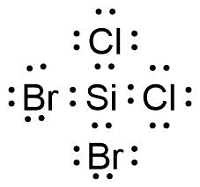# a) Draw the electron-dot formula for SiCl2Br2. Include all lone pairs of electrons. b) Consider...

## Question:

a) Draw the electron-dot formula for SiCl2Br2. Include all lone pairs of electrons.

b) Consider the following four molecules. Which of these satisfy the octet rule and which do not?

i) {eq}PF_{5} {/eq}

ii) {eq}CS_{2} {/eq}

iii) {eq}BBr_{3} {/eq}

iv) {eq}{CO_{3}}^{2-} {/eq}

## Octet Rules

Octet rule states that when atoms in a molecule have eight valence electrons they have added stability and thus it is what atoms in molecules will tend to do. However, substantial exceptions to this rule are observed.

a) the electron dot formula is in the figure below. However, it will be more clear to use lines for bonds.b)

i) {eq}PF_5 {/eq} does not...

Become a Study.com member to unlock this answer! Create your account